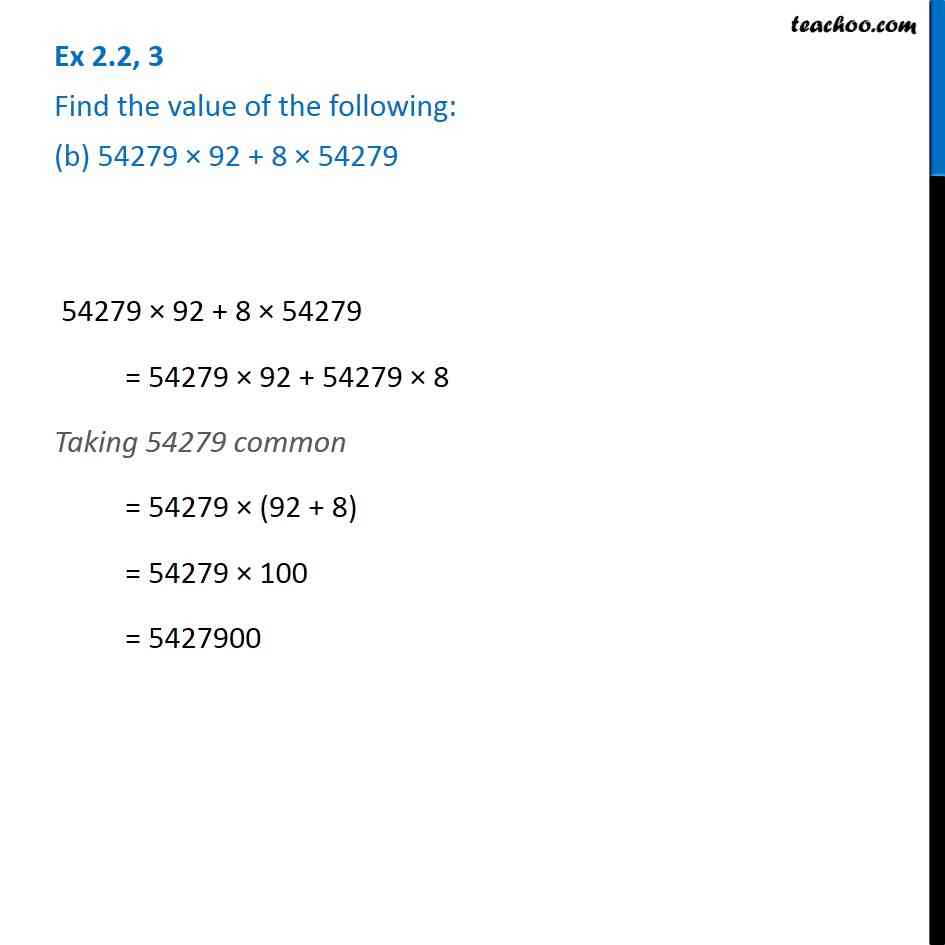Ex 2.2

Chapter 2 Class 6 Whole Numbers
Serial order wiseGet live Maths 1-on-1 Classs - Class 6 to 12

### Transcript

Ex 2.2, 3 Find the value of the following: (b) 54279 × 92 + 8 × 54279 54279 × 92 + 8 × 54279 = 54279 × 92 + 54279 × 8 Taking 54279 common = 54279 × (92 + 8) = 54279 × 100 = 5427900